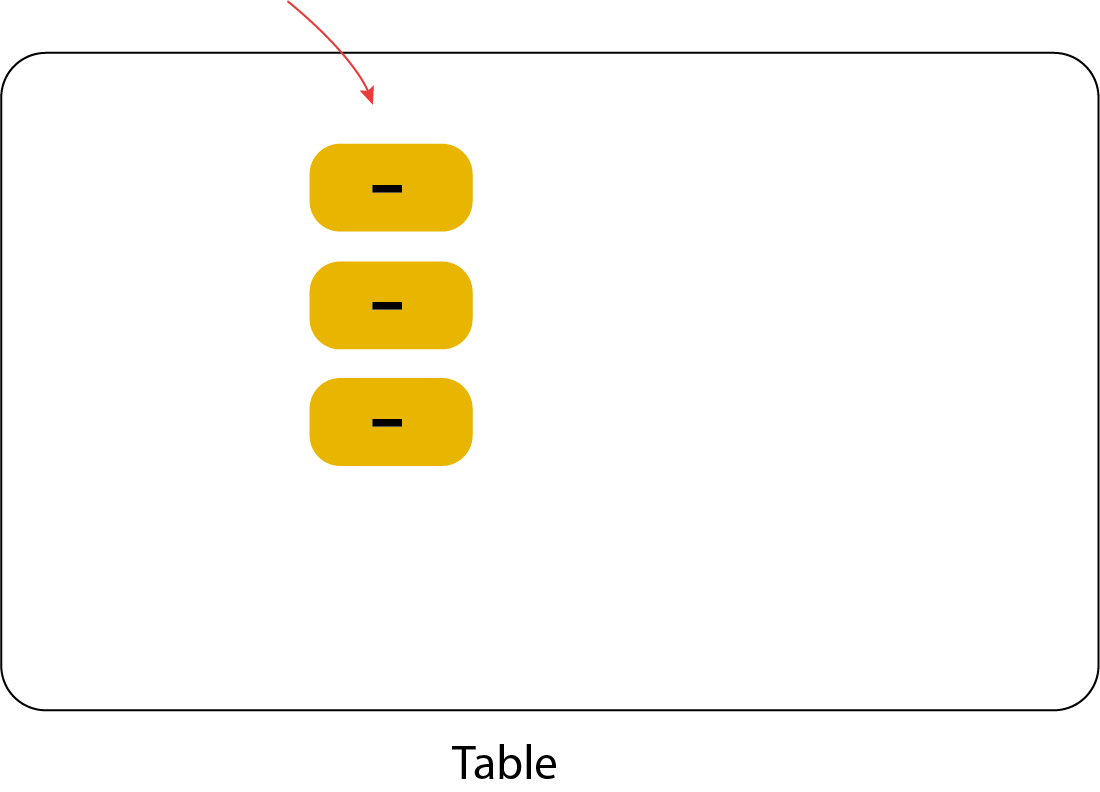### Add and Subtract integers Using Counters

Even though we say addition or subtraction, viewing the operation not as adding or subtracting but rather as tallying good and bad points will enable you to perform your calculations without difficulty.

Before beginning;

Studying our topic on addition - subtraction in integers will significantly aid your understanding of this subject.

Some important key points

1 ) The meaning of the Signs.

Put on the table / Place on the table

Take a way from the table

2) Two Pairs Make Zero

A negative and a positive neutralize each other. They become zero.

# ( +5 ) + ( +3 ) =

i put 5 positive counters on my table.

The middle sign means to put / add  on the table. What are we going to add? Three more positive counters.

8 positive counters on the table.

# ( -2 ) + ( - 5) =

Put 2 negative counters on the table.

Just add 5 more negative counters to the table.

Let's look at the table, what do we have? 7 negative counters !

# ( -6 ) + ( +8) =

We should start by taking 6 negative counters to the table.

My next step is to add, what will I add? 8 positive counters.

Let's see what's on my table? 6 negative counters and 8 positive counters. Remember, 1 negative counter neutralizes 1 positive counter.

There would only be 2 positive counters left on the table, resulting in +2.

# Subtraction

Take a way from table.

# ( +8) - ( +4)

I start by placing 8 positive counters on the table.

I need to take 4 positive counters off the table.

and finally , 4 positive counters are left on the table.

# ( +5) - ( +7) = ?

I start by placing 5 positive counters on the table.

Now I have to take away 7 positive counters, but I don't have 7 counters on the table!

I need to add 2 positive counters to the table. However, if I only add 2 positive counters, I would change the value of the table from 5 to 7. I need the table's value to remain unchanged, but there should also be 7 positive counters on it. You might think this is impossible, but it's not!

If we put one positive and one negative counter on the table, we essentially add zero, meaning we don't change the value of the table.

Now I have 7 positive counters on the table. I can take out the 7 counters.

I have 2 negative counters left on my table.

So the result is ;

example:

# ( - 2 ) - ( +3) = ?

I start by placing 2 negative counters on the table.

Now I have to take away 3 positive counters, but I don't have 3 positive counters on the table!

To get 3 positive counters, I add 3 pairs of positive and negative counters.

so the result is

example;

# ( - 3 ) - ( - 5) = ?

I start by placing 3 negative counters on the table.

#Now I have to take away 5 negative counters, but I don't have 5 negative counters on the table!

To get 5 negative counters, I add 2 pairs of positive and negative counters.

i have 5 negative counters on my table, if I remove 5 negative counters,

2 positive counters are left on my table.